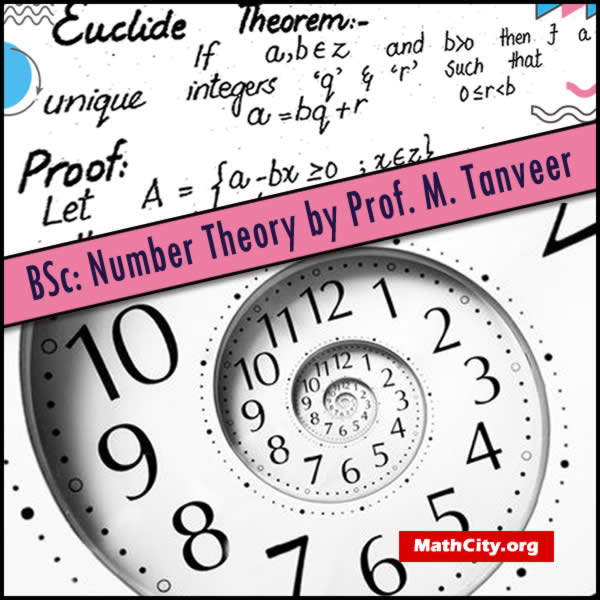# Number Theory by Prof. M. TanveerThese notes are very helpful to prepare one of the sections of mathematics for BSc. Also these notes can be used for other classes.

 Author: Prof. M. Tanveer Handwritten PDF (972 kB) 17
• Divisibility: Let $a,b, \in \mathbb{Z}$, $a \neq 0$. We say that $a$ divides $b$ if there exists $c\in \mathbb{Z}$ such that $b=ac$.
• Common divisor: Let $a,b, \in \mathbb{Z}$, then $c \in \mathbb{Z}$ is called common divisor of $a$ and $b$ if $c | a$ and $c | b$.
• Greatest common divisor (GCD)
• Euclid Theorem
• Least common multiple (LCM)
• Many theorems related to these

• bsc/number-theory-by-prof-m-tanveer